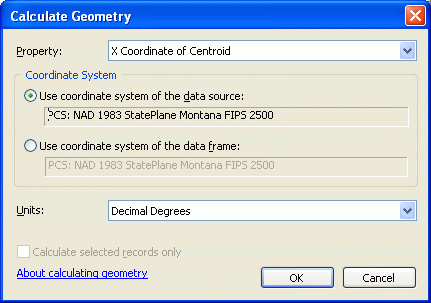# Convert coordinate units using Python

1427
4
03-11-2013 09:35 AM
Status: Implemented
Labels (1)Occasional Contributor II
Add the ability to easily convert the units of XY coordinates using Python code.  For example, using Calculate Geometry tool, I can get the coordinate of a point in meters, feet, decimal degrees, etc.  However, there's no easy way to do this in Python.

The ability to convert units in code is available for areas and lengths.  For example, !shape.length@yards! will calculate the length of a feature in yards.  What about adding a python function like !shape.extent.XMax@decimaldegrees! that will calculate the X coordinate of a point in decimal degrees?
Tags (5)byIt is not that simple because the x coordinate of a point in decimal degrees will be different if the point is in NAD27 vs NAD83. I think the best way to accomplish this is to use the projectAs method on the Geometry object passing in a new spatial reference and appropriate geographic transformation. This will return a new Geometry object and then you can access the X and Y coordinates of the point in the new spatial reference.byChris, I investigated your proposed solution, and it works provided you are working with only geographic coordinate systems. However, working with UTM or MGRS really does not faciliate this.

To relate this solution to be more aligned to existing functinality, all we need is a tool doing the exact same thing as the Convert Coordinate Notation tool, except not requiring a table to be created first. If a method were provided with the same parameters, except the input and output were not a table and feature class, but simply an input set of coordinates and output set of coordinates, this would be ideal.

Right now, to convert in a tool, I have to create an in_memory table with the input coordinates, use the convert coordinate notation tool to convert to another in_memory feature class and extract the SHAPE@X and SHAPE@Y attributes to get what I need. This is a huge amount of overhead for something so simple. A simple method very similar to the functionality provided by the convert coordinate notation tool, except not requiring complete datasets...this is what is needed....yeah but, in the calculate geometry tool you're allowed to enter the Coordinate System to calculate on...byStatus changed to: Implemented

Hi all,

Thank you for your suggestions on this! I'm happy to report that this has since been been implemented with the PointGeometry.toCoordString(<notation>) method, see https://pro.arcgis.com/en/pro-app/2.6/arcpy/classes/pointgeometry.htm

The coordinate string can be turned back into a PointGeometry with the arcpy.fromCoordString(<string>, <notation>) function.

For example:

``````# Create a point in WGS84
point_wgs = arcpy.PointGeometry(
arcpy.Point(-93.109591203, 42.724789892),
arcpy.SpatialReference(4326)
)

# Check result
print(point_wgs.spatialReference.factoryCode)
print(point_wgs)

# Get the coordinates in UTM
utm_coords = point_wgs.toCoordString("UTM")

# Create a new point from UTM coordinates
point_utm = arcpy.FromCoordString(utm_coords, "UTM")

# Check result (note, FromCoordString output always in WGS84)
print(point_utm.spatialReference.factoryCode)
print(point_utm)``````